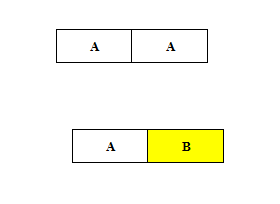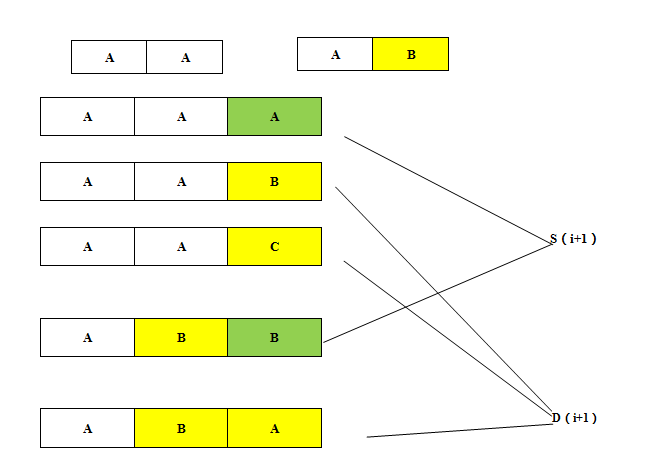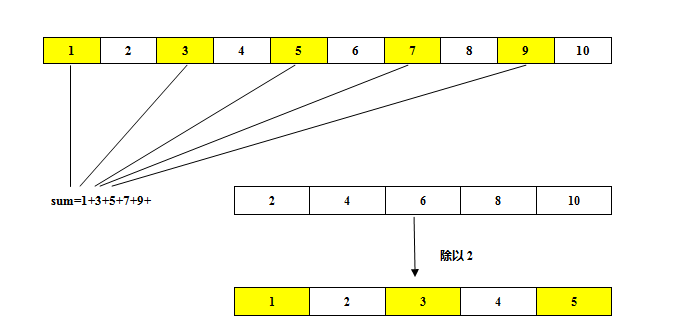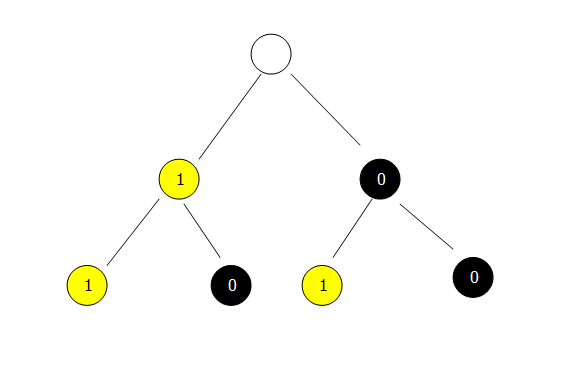# 网易2017秋招编程题集合--完全解析

## 1.回文序列

{1, 2, 1}, {15, 78, 78, 15} , {112} 是回文序列,
{1, 2, 2}, {15, 78, 87, 51} ,{112, 2, 11} 不是回文序列。

4
1 1 1 3

2

package 网易2017秋招编程题集合;

import java.util.Scanner;

public class Main {

public static void main(String[] args) {
Scanner sc=new Scanner(System.in);
while(sc.hasNext())
{
int n=sc.nextInt();
int []arr=new int[n];
for(int i=0;i<n;i++)
arr[i]=sc.nextInt();
getPalindrome(arr);
}
sc.close();
}
public static void getPalindrome(int []arr)
{
int res=0;
int i=0,j=arr.length-1;
while(i<j)
{
//如果首尾相等不处理。
if(arr[i]==arr[j])
{
i++;
j--;
}
//如果首部比尾部小，则与其相邻的加
if(arr[i]<arr[j])
{
arr[i+1]+=arr[i];
i++;
res++;
}
//如果尾部比首部小，则与其相邻的加
if(arr[j]<arr[i])
{
arr[j-1]+=arr[j];
j--;
res++;
}
}
System.out.println(res);
}
}


## 2.优雅的点

25

12

package 网易2017秋招编程题集合;

import java.util.Scanner;

public class Main {

public static void main(String[] args) {
Scanner sc=new Scanner(System.in);
while(sc.hasNext())
{
int r=sc.nextInt();
getElegantPoint(r);
}
sc.close();
}
public static void getElegantPoint(int r)
{
if(r==0)
{
System.out.println(1);
return;
}
int res=0;
double p=Math.pow(r, 0.5);
if(Math.abs(p-Math.ceil(p))<=0.00000001)
{//条件成立则p是一个正整数
res+=4;
}
for(int x=1;x<p;x++)
{
double y=Math.pow(r-x*x, 0.5);
if(Math.abs(y-Math.ceil(y))<=0.00000001)
{
res+=4;
}
}
System.out.println(res);
}

}


## 3.跳石板

N = 4，M = 24：
4->6->8->12->18->24

(4 ≤ N ≤ 100000)
(N ≤ M ≤ 100000)

4 24

5

dp[i+x-N]= Math.min( dp[i+x-N] , dp[i-N] + 1 ); 其中 x 为 i 的公约数。

package 网易2017秋招编程题集合;

import java.util.ArrayList;
import java.util.Arrays;
import java.util.Scanner;

public class Main {

public static void main(String[] args) {
Scanner sc=new Scanner(System.in);
while(sc.hasNext())
{
int N=sc.nextInt();
int M=sc.nextInt();
getstep(N, M);
}
sc.close();
}
//求最小步数
public static void getstep(int N,int M)
{
int dp[]=new int[M-N+1];
Arrays.fill(dp, Integer.MAX_VALUE);
dp=1;
for(int i=N;i<=M;i++)
{
if(dp[i-N]==Integer.MAX_VALUE)
{
continue;
}
ArrayList<Integer> list=getfew(i);
for(int j=0;j<list.size();j++)
{
int x=list.get(j);
if(i+x<=M)
dp[i+x-N]=Math.min(dp[i+x-N], dp[i-N]+1);
}
}
System.out.println(dp[M-N]==Integer.MAX_VALUE?-1:dp[M-N]-1);
}
//求公约数
public static ArrayList<Integer> getfew(int n)
{
ArrayList<Integer> list=new ArrayList<Integer>();
for(int i=2;i*i<=n;i++)
{
if(n%i==0)
{
list.add(i);
if(n/i!=i)
list.add(n/i);
}
}
return list;
}
}


## 4.暗黑的字符串

BAACAACCBAAA 连续子串”CBA”中包含了’A’,’B’,’C’各一个，所以是纯净的字符串
AABBCCAABB 不存在一个长度为3的连续子串包含’A’,’B’,’C’,所以是暗黑的字符串

2
3

9
21package 网易2017秋招编程题集合;

import java.util.Scanner;

public class Main {

public static void main(String[] args) {
Scanner sc=new Scanner(System.in);
while(sc.hasNext())
{
int n=sc.nextInt();
cal(n);
}
sc.close();
}
public static void cal(int n)
{
if(n==1)
{
System.out.println(3);
return;
}
if(n==2)
{
System.out.println(9);
return;
}
long s=3,d=6;
for(int k=3;k<=n;++k)
{
long temp=s;
s=s+d;
d=2*temp+d;
}
System.out.println(s+d);
}
}


## 5.数字翻转

123 100

223

package 网易2017秋招编程题集合;

import java.util.Scanner;

public class Main {

public static void main(String[] args) {
Scanner sc=new Scanner(System.in);
while(sc.hasNext())
{
int x=sc.nextInt();
int y=sc.nextInt();
System.out.println(rev(rev(x)+rev(y)));
}
sc.close();
}
public static int  rev(int n)
{
int res=0;
while(n!=0)
{
res=10*res+(n%10);
n/=10;
}
return res;
}
}


## 6.最大的奇约数

f(1) + f(2) + f(3) + f(4) + f(5) + f(6) + f(7) = 1 + 1 + 3 + 1 + 5 + 3 + 7 = 21

7

21package 网易2017秋招编程题集合;

import java.util.Scanner;

public class Main{

public static void main(String[] args) {
Scanner sc=new Scanner(System.in);
while(sc.hasNext())
{
long n=sc.nextLong();
System.out.println(getMaxOddFew(n));
}
sc.close();
}
public static long getMaxOddFew(long n)
{
long sum=0;
if(n<=1)
return n;
for(int i=1;i<=n;i++)
{
sum+=(i&1)*i;//所有奇数相加。
}
return sum+getMaxOddFew(n/2);
}

}


package 网易2017秋招编程题集合;

import java.util.Scanner;

public class Main {

public static void main(String[] args) {
Scanner sc=new Scanner(System.in);
while(sc.hasNext())
{
long n=sc.nextLong();
System.out.println(getMaxOddFew(n));
}
sc.close();
}
public static long getMaxOddFew(long n)
{
long sum=0;
if(n<=1)
return n;
if((n&1)==1)
{
sum=(n+1)*(n+1)/4;
}
else {
sum=n*n/4;
}
//使用数学公式计算奇数和，大大减小了时间复杂度。
return sum+getMaxOddFew(n/2);
}

}


## 7.买苹果

20

3package 网易2017秋招编程题集合;

import java.util.Arrays;
import java.util.Scanner;

public class Main {
static int min=Integer.MAX_VALUE;
public static void main(String[] args) {
Scanner sc=new Scanner(System.in);
while(sc.hasNext())
{
int n=sc.nextInt();
getapple(n);
}
sc.close();
}
public static void getapple(int n)
{
min=Integer.MAX_VALUE;
getapple(n,0);
System.out.println(min==Integer.MAX_VALUE?-1:min);
}
public static void getapple(int n,int count)
{
if(n<=0)
{
if(n==0)
min=Math.min(count, min);
return;
}
getapple(n-6,count+1);//遍历左子树
getapple(n-8,count+1);//遍历右子树
}
}


if(i+6<=n)

dp[i+6]=Math.min(dp[i+6], dp[i]+1);

if(i+8<=n)

dp[i+8]=Math.min(dp[i+8], dp[i]+1);

public static void getapple_dp(int n)
{
int []dp=new int[n+1];
Arrays.fill(dp,Integer.MAX_VALUE);
dp=0;
for(int i=0;i<=n;i++)
{
if(dp[i]==Integer.MAX_VALUE)
continue;
if(i+6<=n)
dp[i+6]=Math.min(dp[i+6], dp[i]+1);
if(i+8<=n)
dp[i+8]=Math.min(dp[i+8], dp[i]+1);
}
System.out.println(dp[n]==Integer.MAX_VALUE?-1:dp[n]);
}

## 8.计算糖果

**题目描述：**A,B,C三个人是好朋友,每个人手里都有一些糖果,我们不知道他们每个人手上具体有多少个糖果,但是我们知道以下的信息：
A - B, B - C, A + B, B + C. 这四个数值.每个字母代表每个人所拥有的糖果数.

1 -2 3 4

2 1 3

package 网易2017秋招编程题集合;

import java.util.Scanner;

public class Main {

public static void main(String[] args) {
Scanner sc=new Scanner(System.in);
while(sc.hasNext())
{
int a_b=sc.nextInt();
int b_c=sc.nextInt();
int ab=sc.nextInt();
int bc=sc.nextInt();
getABC(a_b, b_c, ab, bc);
}
sc.close();
}
public static void getABC(int a_b,int b_c,int ab,int bc)
{
int a=(a_b+ab)/2;
int b=(b_c+bc)/2;
int c=bc-b;
if(a-b==a_b&&(b-c==b_c)&&(a+b==ab)&&(b+c==bc))
{
System.out.println(a+" "+b+" "+c);
}
else {
System.out.println("No");
}
}
}

12-03447
11-081万+03-21278
09-22670
02-28379
08-151865
07-091534
09-08252
01-253693
12-172224
02-02737
10-04538
08-151830
09-29
04-137358
04-08995
©️2020 CSDN 皮肤主题: Age of Ai 设计师:meimeiellie